• K-means++
千次阅读 热门讨论
2022-06-29 21:55:30

​前 言：作为当前先进的深度学习目标检测算法YOLOv5，已经集合了大量的trick，但是还是有提高和改进的空间，针对具体应用场景下的检测难点，可以不同的改进方法。此后的系列文章，将重点对YOLOv5的如何改进进行详细的介绍，目的是为了给那些搞科研的同学需要创新点或者搞工程项目的朋友需要达到更好的效果提供自己的微薄帮助和参考。

解决问题：YOLOv5默认采用K-Means算法聚类COCO数据集生成的锚框，并采用遗传算法在训练过程中调整锚框，但是K-Means在聚类时，从其算法的原理可知，K-Means正式聚类之前首先需要完成的就是初始化k个簇中心。同时，也正是因为这个原因，使得K-Means聚类算法存在着一个巨大的缺陷——收敛情况严重依赖于簇中心的初始化状况，采用K-Means++可以有效缓解这一问题，从而一定程度上能够提高检测精度和效果。

原理：

K-Means++算法实际就是修改了K-Means算法的第一步操作之所以进行这样的优化，是为了让随机选取的中心点不再只是趋于局部最优解，而是让其尽可能的趋于全局最优解。要注意“尽可能”的三个字，即使是正常的K-Means++算法也无法保证百分百全局最优，在说取值原理之后我们就能知道为什么了思路就是我们要尽可能的保证各个簇的中心点的距离要尽可能的远当簇的中心尽可能的远的时候就能够尽可能的保证中心点之间不会在同一个簇内K-Means的迭代实际上就是簇的形状的修改，只要初始形状不太出格就会回归于正确形状。

方 法：

运行下面的程序可以得到K-Mean++聚类生成的锚框。部分代码如下。

from __future__ import division, print_function

import numpy as np
import random
import math

def iou(box, clusters):
x = np.minimum(clusters[:, 0], box)
y = np.minimum(clusters[:, 1], box)
if np.count_nonzero(x == 0) > 0 or np.count_nonzero(y == 0) > 0:
raise ValueError("Box has no area")

intersection = x * y
box_area = box * box
cluster_area = clusters[:, 0] * clusters[:, 1]

iou_ = np.true_divide(intersection, box_area + cluster_area - intersection + 1e-10)
# iou_ = intersection / (box_area + cluster_area - intersection + 1e-10)

return iou_

def iou_kpp(box, clusters):
x = np.minimum(clusters, box)
y = np.minimum(clusters, box)
if np.count_nonzero(x == 0) > 0 or np.count_nonzero(y == 0) > 0:
raise ValueError("Box has no area")

intersection = x * y
box_area = box * box
cluster_area = clusters * clusters

iou_ = np.true_divide(intersection, box_area + cluster_area - intersection + 1e-10)
# iou_ = intersection / (box_area + cluster_area - intersection + 1e-10)

return iou_

def avg_iou(boxes, clusters):
return np.mean([np.max(iou(boxes[i], clusters)) for i in range(boxes.shape)])

def get_closest_dist(point, centroids):
min_dist = math.inf  # 初始设为无穷大
# print(centroids)
for i, centroid in enumerate(centroids):
# print(centroids)
dist = 1 - iou_kpp(point, centroid)		# 点和当前每个中心点进行计算距离
if dist < min_dist:
min_dist = dist		# 注意我K-means++博客中的这句“指该点离中心点这一数组中所有中心点距离中的最短距离”
return min_dist

def kpp_centers(data_set: list, k: int) -> list:
"""
从数据集中返回 k 个对象可作为质心
"""
cluster_centers = []
cluster_centers.append(random.choice(data_set))
d = [0 for _ in range(len(data_set))]
#print(d)
for _ in range(1, k):
total = 0.0
for i, point in enumerate(data_set):
d[i] = get_closest_dist(point, cluster_centers) # 与最近一个聚类中心的距离
total += d[i]
total *= random.random()
for i, di in enumerate(d): # 轮盘法选出下一个聚类中心；
total -= di
if total > 0:
continue
cluster_centers.append(data_set[i])
break
return cluster_centers

def kmeans(boxes, k, dist=np.median):
rows = boxes.shape

last_clusters = np.zeros((rows,))

np.random.seed()

# the Forgy method will fail if the whole array contains the same rows
clusters = kpp_centers(boxes, k)
clusters = np.array(clusters)
#clusters = boxes[np.random.choice(rows, k, replace=False)] 这是K-means的，两个切换注释下就行了

while True:
for row in range(rows):
distances[row] = 1 - iou(boxes[row], clusters)  # iou很大则距离很小
# 对每个标注框选择与其距离最接近的集群中心的标号作为所属类别的编号。
nearest_clusters = np.argmin(distances, axis=1)     # axis=1表示沿着列的方向水平延伸

if (last_clusters == nearest_clusters).all():
break

for cluster in range(k):
clusters[cluster] = dist(boxes[nearest_clusters == cluster], axis=0)    # 给每类算均值新中心点
# print(last_clusters)

return clusters

def parse_anno(annotation_path, target_size=None):
anno = open(annotation_path, 'r',encoding='utf-8')
result = []
# 对每一个标记图片
for line in anno:
s = line.strip().split(' ')
img_w = int(s)
img_h = int(s)
s = s[3:]
box_cnt = len(s) // 5
# 分别处理每一个标记框的信息，并提取标记框的高度和宽度，存入result 列表
for i in range(box_cnt):
x_min, y_min, x_max, y_max = float(s[i*5+1]), float(s[i*5+2]), float(s[i*5+3]), float(s[i*5+4])
width = x_max - x_min
height = y_max - y_min
# assert width > 0
# assert height > 0
# use letterbox resize, i.e. keep the original aspect ratio
# get k-means anchors on the resized target image size
if target_size is not None:
resize_ratio = min(target_size / img_w, target_size / img_h)
width *= resize_ratio
height *= resize_ratio
result.append([width, height])
# get k-means anchors on the original image size
else:
result.append([width, height])
result = np.asarray(result)
return result

def get_kmeans(anno, cluster_num=9):
# 使用kmeans算法计算需要的anchors
anchors = kmeans(anno, cluster_num)

ave_iou = avg_iou(anno, anchors)
# 格式化为int类型
anchors = anchors.astype('int').tolist()
# 按照面积大小排序，
anchors = sorted(anchors, key=lambda x: x * x)

return anchors, ave_iou

if __name__ == '__main__':

# ave_iou=0.1
n=12

target_size = [640, 640]
annotation_path = "diorship-kmean++_test.txt"
anno_result = parse_anno(annotation_path, target_size=target_size)
anchors, ave_iou = get_kmeans(anno_result, n)

# 格式化输出anchors数据
anchor_string = ''
for anchor in anchors:
anchor_string += '{},{}, '.format(anchor, anchor)
anchor_string = anchor_string[:-2]

print('anchors are:')
print(anchor_string)
print('the average iou is:')
print(ave_iou)
# while n<14:
#     target_size = [640, 640]
#     annotation_path = "gongjingkmean++_train.txt"
#     anno_result = parse_anno(annotation_path, target_size=target_size)
#     anchors, ave_iou = get_kmeans(anno_result, n)
#
#     # 格式化输出anchors数据
#     anchor_string = ''
#     for anchor in anchors:
#         anchor_string += '{},{}, '.format(anchor, anchor)
#     anchor_string = anchor_string[:-2]
#     print('n is')
#     print(n)
#     print('anchors are:')
#     print(anchor_string)
#     print('the average iou is:')
#     print(ave_iou)
#     # if n==11 :
#     #     n=n+2
#     # else:
#     n=n+1

结 果：本人在多个数据集上做了大量实验，针对不同的数据集效果不同，有轻微的提升作用。下面的图为在遥感数据集上聚类的两种算法的对比图。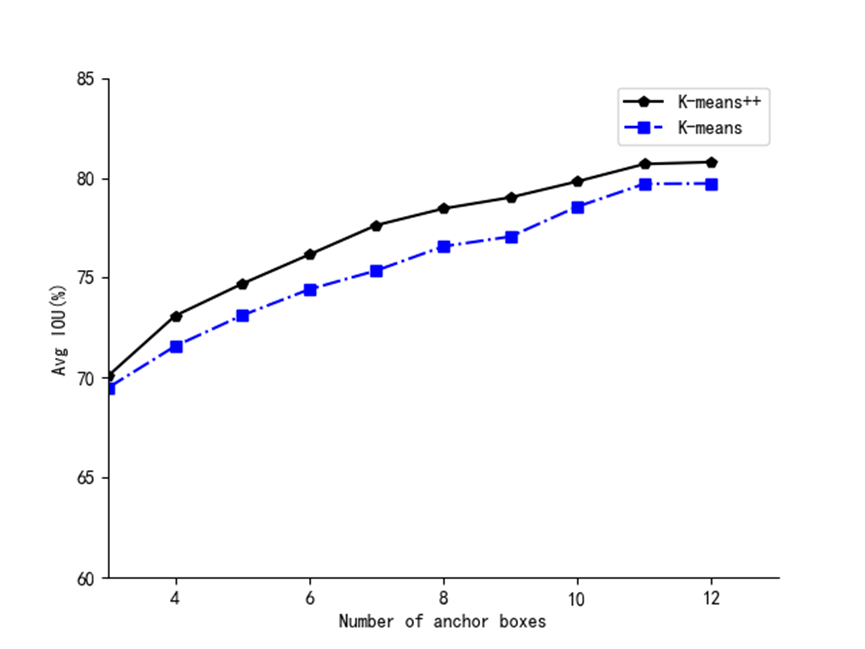从图中可知K-Means++能够聚类出更好的先验框。

预告一下：下一篇内容分享损失函数的优化方法——将EIOU改进为SIOU。有兴趣的朋友可以关注一下我，有问题可以留言或者私聊我哦！

PS：K-Means算法的改进得到的锚框不仅仅是适用改进YOLOv5，也可以改进其他需要锚框检测算法，比如YOLO网络YOLOv4、v3等。

最后，希望能互粉一下，做个朋友，一起学习交流。大数据
更多相关内容
• 此实现解决了使用k-means ++算法的任意初始化方法的一些缺点（请参见最后的“更多阅读”）。 安装 通过npm安装 通过NPM将kmpp安装为Node.js模块： \$ npm install kmpp 例子 var kmpp = require ( 'kmpp' ) ; kmpp ...
• K-Means和K-Means++算法的数据集。包含了两个特征的数据集，分别为XOY坐标轴中的X坐标和Y坐标。不带有类别标签。数据集
• kmeans算法的一种改进算法，叫做kmeans++算法； 英文版； 超级容易懂的说明~
• 用于聚类多元数据的 k-means++ 算法的有效实现。 已经表明，该算法具有对 log(k) 竞争的总簇内距离的期望值的上限。 此外，k-means++ 通常比普通 k-means 收敛得多。matlab
• k-means算法进阶版本k-means++，能够实现结果统计并可视化
• 基于Matlab实现： 模式识别 改进的K-Means++算法 实现模式分类
• 最后针对K-means的缺点，运用改进K-means （K-means++）和肘部法则对RFMT模型进行聚类分析.该模型能对客户群进行更加细致的划分，既能帮助电子商务企业识别出需要重点关注的客户即已流失客户和新客户群体，同时将该...
• ## K-Means及K-Means++算法Python源码实现

千次阅读 多人点赞 2021-05-31 23:51:22
K-Means及K-Means++算法Python源码实现

# 1. K-Means 基本理论

## 1.1 距离度量

聚类（clustering）的基本思想：根据物以类聚的原理，把数据分成不同的组或类，使得组与组之间的相似度尽可能小，而组内数据之间具有较高的相似度。聚类又被称为无监督学习（unsupervised-learning）。

聚类的目标是使得聚类内部对象之间的距离尽可能的小，或者说使它们之间具有很高的相似度。在聚类算法中，一般用距离函数来定义相似度。常用的距离度量包括：

1. 欧氏距离：定义了多维空间中点与点之间的直线距离。
1. 曼哈顿距离：亦称为街区距离，它是在多维空间内从一个对象到另一个对象的折线距离。
1. 切比雪夫距离：类似于从国际象棋的棋盘中的一点走到另一点的最少步数。
1. 余弦相似度：用向量空间中两个向量夹角的余弦值衡量两个个体间的差异的大小。
1. 兰氏距离：消除了量纲，克服了闵可夫斯基距离需要保持各维度指标在相同时刻级别的限制。它受离群值影响较小，适合于数据具有高度偏移的应用。
1. 汉明距离：用来表示两个同等长度的字符串，由一个转换为另一个的最小替换次数。

## 1.2 评估指标

• 轮廓分数（Silhouette Score）：在不了解样本标签的情况下，评估聚类算法性能的最常用方法。它提供了每个样本索引和全局图形表示，显示了聚类的内部一致性和分离水平。
• 调整的兰德系数（ARI）：取值范围在[-1,1]，值越大意味着聚类结果与真实情况相吻合。
• 互信息评分（MI）：可以利用互信息衡量实际类别与预测类别的吻合程度。
• 同质性、完整性和调和平均：范围为[0,1]，值越大代表着聚类效果与真实情况越吻合。
• 轮廓系数：轮廓系数是所有样本轮廓系数的平均值。轮廓系数的范围为[-1,1]，同类别样本距离越近且不同类别样本距离越远，分数越高。
• Calinski-Harabz指数：簇之间的协方差越大，簇与簇之间界限越明显，聚类效果也就越好。簇内部数据的协方差越小，同一个簇内包含的样本越相似，聚类效果越好。

## 1.3 K-Means## 1.3 Mini-Batch K-Means

小批量K均值算法不使用所有的数据样本，而是每次从不同聚类的样本中随机选取一部分样本来代表各自的聚类进行计算，所以精度会有损失，但是速度上会有提升。source: sklearn examplesource:geeksforgeeks

## 1.4 K-Means ++

K-means 是最常用的基于欧式距离的聚类算法，其认为两个目标的距离越近，相似度越大。其核心思想是：首先随机选取k个点作为初始局累哦中心，然后计算各个对象到所有聚类中心的距离，把对象归到离它最近的的那个聚类中心所在的类。重复以上过程，直到达到终止条件。

K-means算法得到的聚类结果严重依赖与初始簇中心的选择，如果初始簇中心选择不好，就会陷入局部最优解，因此提出了K-means++算法，它改进了K-means算法初始中心点的选取，其核心思想是：再选择一个新的聚类中心时，距离已有聚类中心越远的点，被选取作为聚类中心的概率越大。

# 2. K-Means及K-Means++源码实现

完整代码如下，自定义模块从github下载即可。

import sys
import numpy as np
import pandas as pd
from copy import deepcopy
from matplotlib import pyplot as plt

from DistanceMetric import calculate_distance_multi_dims, calculate_distance
from PlotFunctions import plot_cluster_data, generate_colors, plot_k_means_centroids, plot_cluster_process_2d, plot_cluster_result_2d

def do_init_centroids(X, k, method='random'):
"""
初始化聚类中心
----
X: array, dataset
k: int, cluster number
method: str, 'random'(k-means); 'k-means++'
----
KMeans++改进了KMeans算法选择初始质心的方式。
其核心思想是：在选择一个聚类中心时，距离已有的聚类中心越远的点，被选取作为聚类中心的概率越大。
"""
# 样本个数
n_samples  = X.shape
# 样本特征数
n_features = X.shape

# 生成样本索引
indexs = np.arange(0, n_samples)
# 打乱顺序
# 此函数仅沿多维数组的第一个轴对数组进行打乱。子数组的顺序改变，但内容不变。
np.random.shuffle(indexs)
# 初始化聚类簇中心，shape=(k, n_features)
centroids = np.zeros((k, n_features))

# 类型转换，统一格式为 numpy.array
if type(X) == pd.core.frame.DataFrame:
X = X.to_numpy()

if method == 'k-means++':
# 从数据集中随机选择一个样本点作为第一个聚类中心
centroids[0, ] = X[indexs, :]
print(centroids.shape)

# 从剩余样本中选择 k - 1 个聚类中心
for centroid in range(k - 1):
# 定义一个列表存储离聚类中心最近的样本点
dists = []

for i in range(n_samples):
# 单一样本
point = X[i, :]
# 初始化距离
min_dist = sys.maxsize

# 计算 point 与之前的每一个聚类中心的距离
# 选择质心并存储最小距离
for j in range(len(centroids)):
# temp_dist = calculate_distance_multi_dims(point, centroids[j], axis=0)
temp_dist = calculate_distance(point, centroids[j], method='euclidean', p=None)
# 存储最小距离
min_dist = min(min_dist, temp_dist)

dists.append(min_dist)

# 遍历完样本之后，选择距离最大的数据点作为下一个质心
max_dist = np.argmax(np.array(dists))
next_centroid = X[max_dist, :]
# 存储第二个及其之后的聚类中心
centroids[centroid+1, :] = next_centroid

# dists 清零
dists = []

# 随机初始化：即随机从样本中选择 k 个样本点作为初始聚类中心
else:
# 取打乱顺序之后的前 k 个样本作为初始聚类中心
top_k_index = indexs[:k]
# 用这k个样本的值作为初始化的簇中心
centroids = X[top_k_index, :]

return centroids

def k_means(X, n_cluster, init_method='random', n_iter=100, plot_process=False):
init_centroids = do_init_centroids(X, k=n_cluster, method=init_method)
#     print(init_centroids.shape)

# 类型转换，统一格式为 numpy.array
if type(X) == pd.core.frame.DataFrame:
X = X.to_numpy()

# 用于保存聚类中心更新前的值
old_centroids = np.zeros(init_centroids.shape)
#     print(old_centroids.shape)

# 更新后的聚类中心的值
new_centroids = deepcopy(init_centroids)

# 用于保存数据所属的簇
n_samples = len(X)
clusters = np.zeros(n_samples)

# 迭代标识符，计算新旧聚类中心的距离
distance_flag = calculate_distance_multi_dims(init_centroids, old_centroids, axis=1)

if n_iter:
current_iter = 1
iteration_flag = (current_iter < n_iter)
# 去掉最大循环次数限制
else:
iteration_flag = True

# 若聚类中心不再变化或者迭代次数超过n_iter次(可取消)，则退出循环
while distance_flag.any() != 0 and iteration_flag:
# 1. 计算每个样本点所属的簇（距离最近的簇）
for i in range(n_samples):
# 样本与k个聚类中心的距离
distances = calculate_distance_multi_dims(X[i], new_centroids, axis=1)
# 当前样本与k个聚类中心的最近距离
cluster = np.argmin(distances)
# 记录当前样本点所属的聚类中心
clusters[i] = cluster

# 2. 更新聚类中心
# 记录更新前的聚类中心
old_centroids = deepcopy(new_centroids)

# 属于同一个簇的样本点放到一个数组中，然后按照列的方向取平均值
for i in range(n_cluster):
points = [X[j] for j in range(len(X)) if clusters[j] == i]
new_centroids[i] = np.mean(points, axis=0)

# 3. 判断是否满足迭代停止条件
if current_iter % 5 == 0:
print(f"[INFO] Iteration {current_iter}：distance_flag = {distance_flag}.")

distance_flag = calculate_distance_multi_dims(new_centroids, old_centroids, axis=1)
current_iter += 1

if plot_process:    # 如果绘制图像
plt = plot_cluster_process_2d(X, new_centroids,old_centroids) # 画聚类中心的移动过程

if plot_process:    # 显示最终的绘制结果
plt.show()

# 返回每个样本所属的类以及更新后的聚类中心
return clusters, new_centroids

if __name__ == '__main__':
from sklearn.datasets import make_blobs
X, y = make_blobs(n_samples=800, n_features=3, centers=4)
# Importing the dataset
print(data.shape)

plot_cluster_data(data)
plot_cluster_data(X, show_dims=3)

# K-Means
centroids = do_init_centroids(X, k=3, method='random')
print(centroids)
plot_k_means_centroids(X, centroids)
clusters, centroids = k_means(X, n_cluster=3, init_method='random', n_iter=100, plot_process=True)
plot_cluster_result_2d(X, clusters, centroids)

# K-Means++
centroids = do_init_centroids(X, k=3, method='k-means++')
print(centroids)
plot_k_means_centroids(X, centroids)
clusters, centroids = k_means(X, n_cluster=3, init_method='k-means++', n_iter=100, plot_process=True)
plot_cluster_result_2d(X, clusters, centroids)
# skelarn K-MEANS
from sklearn.cluster import KMeans

# Number of clusters
kmeans = KMeans(n_clusters=3)
# Fitting the input data
kmeans = kmeans.fit(X)
# Getting the cluster labels
labels = kmeans.predict(X)
# Centroid values
centroids = kmeans.cluster_centers_

# Comparing with scikit-learn centroids
print("Centroid values")
print("Scratch")
print(centroids) # From Scratch
print("sklearn")
print(centroids) # From sci-kit learn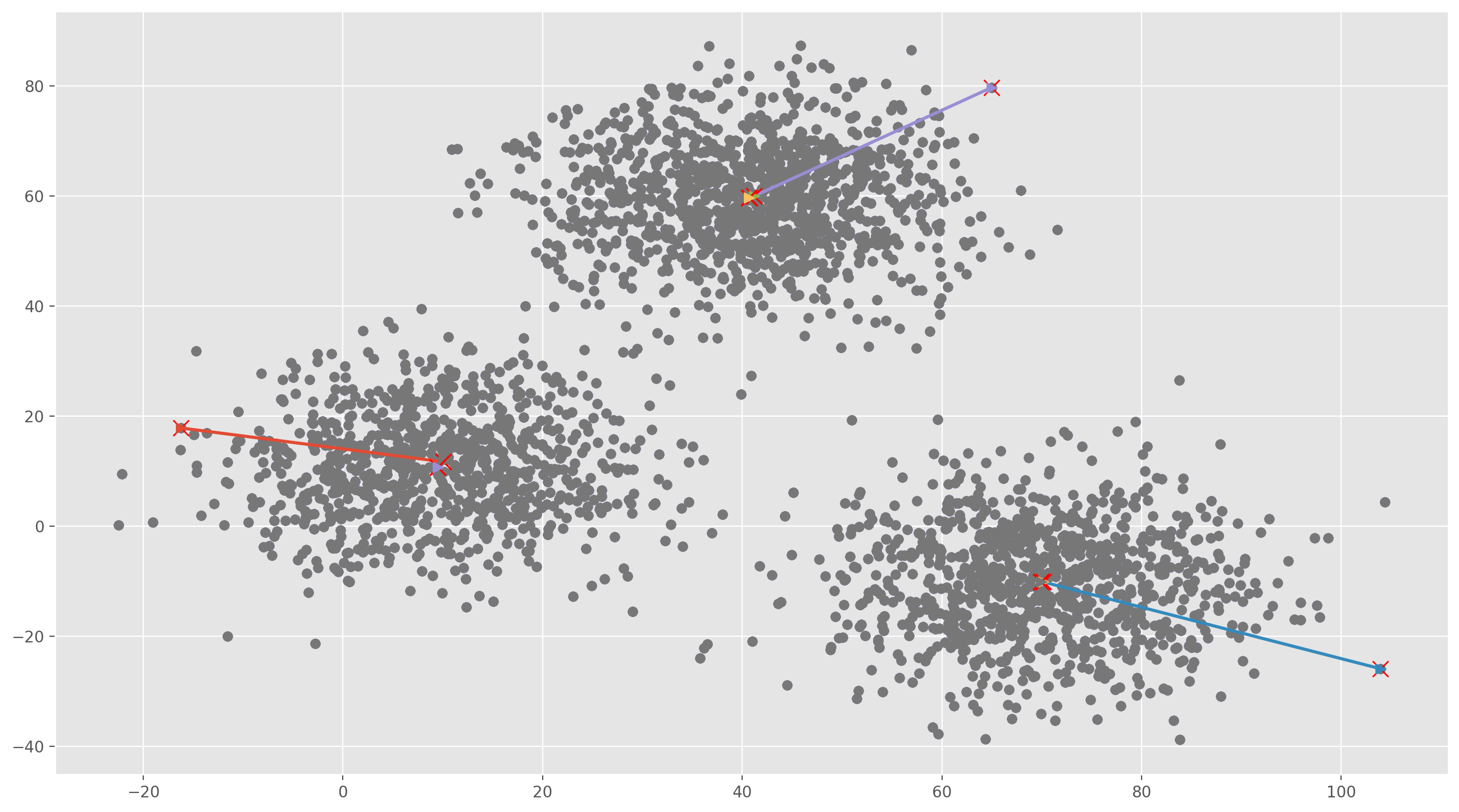聚类中心移动过程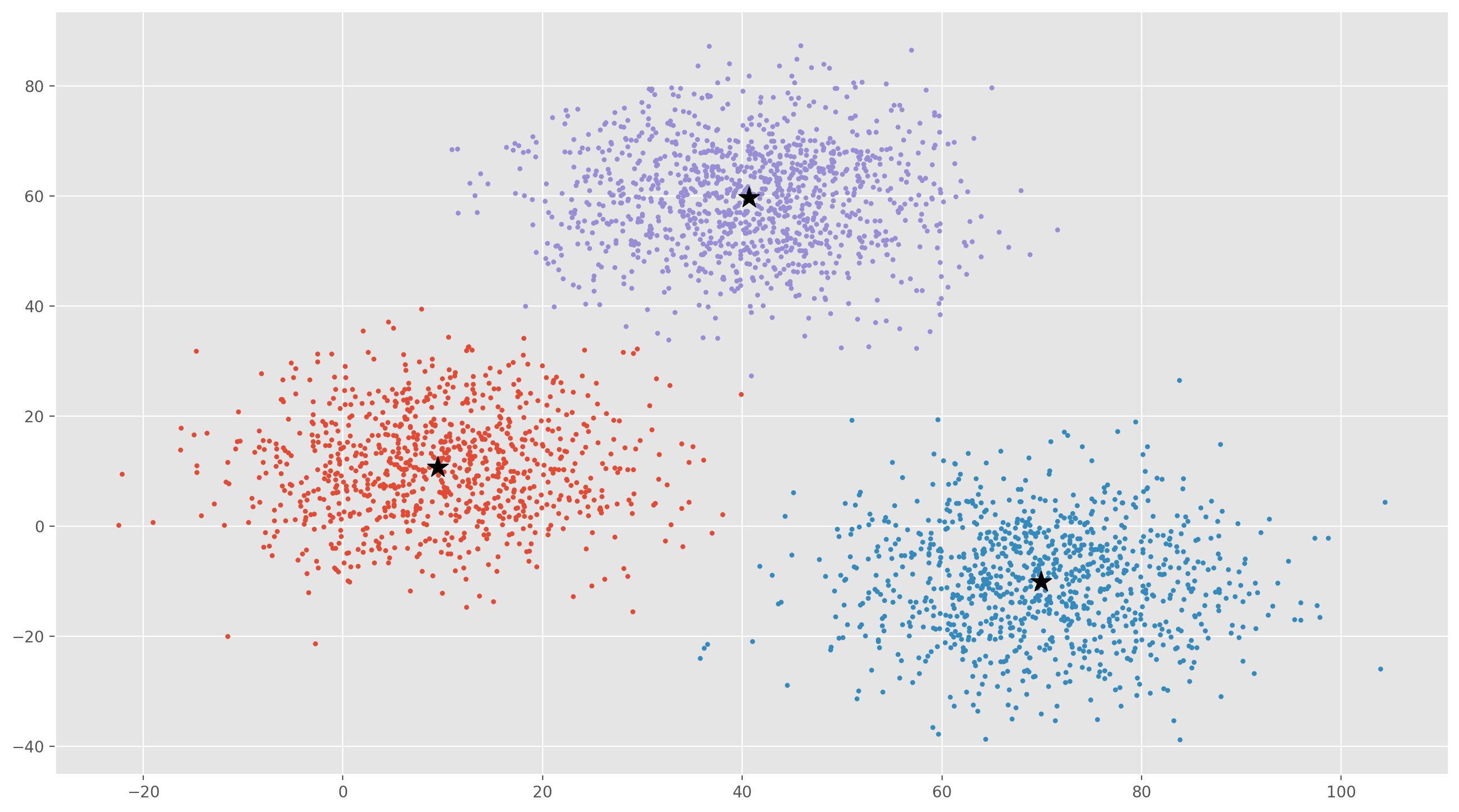聚类结果

# 3. K-Means sklearn实现

数据集获取：

• xclara.csv: https://www.kaggle.com/hdriss/xclara

## 3.1 K-Means

构造数据集

import numpy as np
from sklearn.datasets import make_classification
import matplotlib.pyplot as plt
plt.rcParams['figure.dpi'] = 300

# define dataset
X, y = make_classification(n_samples=1000,
n_features=2,
n_informative=2,
n_redundant=0,
n_clusters_per_class=1, random_state=4)
print(X.shape,y.shape)

for class_value in range(2):
row_ix = np.where(y == class_value)
plt.scatter(X[row_ix, 0], X[row_ix, 1], label=class_value)

plt.xlabel('Feature 1')
plt.ylabel('Feature 2')
plt.legend()
plt.show()

from sklearn.cluster import KMeans

model = KMeans(n_clusters=2, init='random')
model.fit(X)
yhat = model.predict(X)
clusters = np.unique(yhat)

for cluster in clusters:
row_ix = np.where(yhat == cluster)
plt.scatter(X[row_ix, 0], X[row_ix, 1], label=cluster)

plt.xlabel('Feature 1')
plt.ylabel('Feature 2')
plt.legend(ncol=2)
plt.show()


## 3.2 K-Means ++

model = KMeans(n_clusters=2, init='k-means++')
model.fit(X)
yhat = model.predict(X)
clusters = np.unique(yhat)

for cluster in clusters:
row_ix = np.where(yhat == cluster)
plt.scatter(X[row_ix, 0], X[row_ix, 1], label=cluster)

plt.xlabel('Feature 1')
plt.ylabel('Feature 2')
plt.legend(ncol=2)
plt.show()


## 3.3 Mini-Batch K-Means

from sklearn.cluster import MiniBatchKMeans, KMeans
from sklearn.metrics.pairwise import pairwise_distances_argmin
from sklearn.datasets import make_blobs

model = KMeans(n_clusters=2, init='k-means++')
model.fit(X)
yhat = model.predict(X)
clusters = np.unique(yhat)

for cluster in clusters:
row_ix = np.where(yhat == cluster)
plt.scatter(X[row_ix, 0], X[row_ix, 1], label=cluster)

plt.xlabel('Feature 1')
plt.ylabel('Feature 2')
plt.legend(ncol=2)
plt.show()


# 4. 聚类中心数量 k 的选取

cost =[]
for i in range(1, 11):
#     km = k_means(data, n_cluster=i, init_method='k-means++',
#                  n_iter=100, plot_process=True)
KM = KMeans(n_clusters = i, max_iter = 500)
KM.fit(X)
# calculates squared error for the clustered points
cost.append(KM.inertia_)

# plot the cost against K values
plt.plot(range(1, 11), cost, color ='g', linewidth ='3')
plt.xlabel("Value of K")
plt.ylabel("Sqaured Error (Cost)")
plt.show() # clear the plot


# Reference

1. https://www.cnblogs.com/wj-1314/p/12751033.html
2. https://www.cnblogs.com/shenfeng/p/kmeans_demo.html
3. https://mubaris.com/posts/kmeans-clustering/
4. https://github.com/lawlite19/MachineLearning_Python/blob/master/K-Means/K-Menas.py
5. https://www.geeksforgeeks.org/ml-k-means-algorithm/
6. https://www.geeksforgeeks.org/ml-mini-batch-k-means-clustering-algorithm/
展开全文机器学习 聚类 python kmeans算法
• ( 2007_k-means++, the advantages of careful seeding论文
• K-means++算法： K-means++算法是基于传统K-means的改进版，改进的地方在于，它的聚类中心不是随机产生的，而是在一开始就已经通过一种十分有效的方式给选出来了,但是有一点仍旧是需要给出初始的第一个聚类中心，第...

经典Kmeans算法是最常用的一种聚类算法。感觉在西瓜书里面最容易看懂的，而且最容易用的一个算法便是k-mean算法，算法实现的流程十分简单，可以简单将其划分为4个步骤：

Step1:选定聚类中心，从数据集中随机选取K个样本作为初始聚类中心，{}, i = 1, 2, 3, 4, 5, ... n ;

Step2:计算欧式距离，并对其进行归类，遍历数据集中的每一个样本，计算每一个样本与所有初始聚类中心的欧式距离，将其加入到欧式距离最小的那个聚类中心的一类中

Step3:更新聚类中心，重新计算每一类的中心, n每一个类中的样本个数

Step4: 重新聚类，重新遍历数据集中的每一个样本，计算每一个样本与所有新的聚类中心的欧式距离，将其加入到欧式距离最小的那个聚类中心的一类中

不断重复上面四个步骤，直至聚类中心不再改变，聚类停止。K-means++算法：

K-means++算法是基于传统K-means的改进版，改进的地方在于，它的聚类中心不是随机产生的，而是在一开始就已经通过一种十分有效的方式给选出来了,但是有一点仍旧是需要给出初始的第一个聚类中心，第一个聚类中心是随机产生的。

对K-means++算法原理进行简单总结一下：

首先，其实的簇中心是我们通过在样本当中随机得到的。不过我们并不是一次性随机K个，而是只随机1个。

接着，我们要从生下的n-1个点当中再随机出一个点来做下一个簇中心。但是我们的随机不是盲目的，我们希望设计一个机制，使得距离所有簇中心越远的点被选中的概率越大，离得越近被随机到的概率越小

我们重复上述的过程，直到一共选出了K个簇中心为止。

K-menas++便是针对这个机制提出了一个有效的方法，比较简单的称为轮盘法，下面简单介绍一下：

我们来看一下如何根据权重来确定概率，实现这点的算法有很多，其中比较简单的是轮盘法。这个算法应该源于赌博或者是抽奖，原理也非常相似。

我们或多或少都玩过超市或者是其他场景下的转盘抽奖，在抽奖当中有一个指针一直保持不动。我们转动转盘，当转盘停下的时候，指针所指向的位置就是抽奖的结果。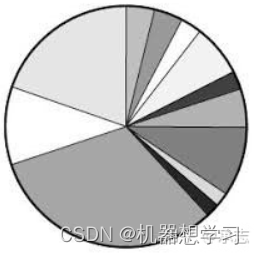我们都知道命中结果的概率和轮盘上对应的面积有关，面积越大抽中的概率也就越大，否则抽中的概率越小。

对于每一个点，被选中的概率为：

其中是每个点到所有类簇的最短距离，表示点被选中作为类簇中心的概率。

轮盘法其实就是一个模拟转盘抽奖的过程，只不过我们用数组模拟了转盘。我们把转盘的扇形拉平，拉成条状，原来的每个扇形就对应了一个区间。扇形的面积就对应了区间的长度，显然长度越长，抽中的概率越大。然后我们来进行抽奖，我们用区间的长度总和乘上一个0-1区间内的数。

我们找到这个结果落在的区间，就是这次轮盘抽中的结果。这样我们就实现了控制随机每个结果的概率。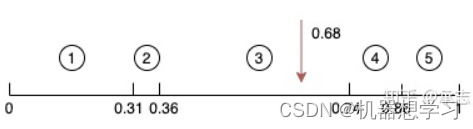在上面这张图当中，我们随机出来的值是0.68，然后我们一次减去区间，最后落到的区间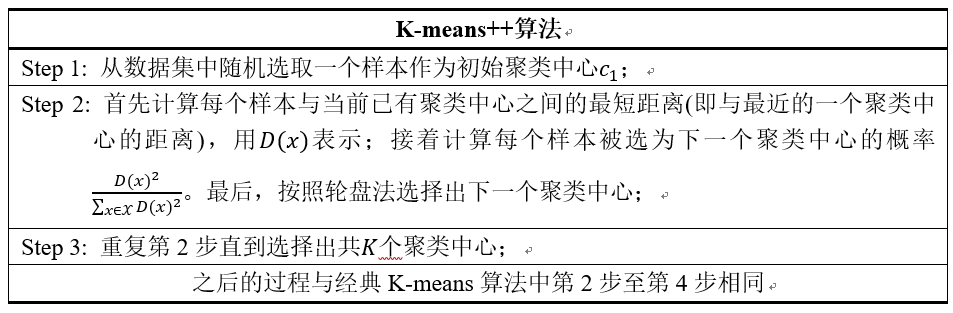上面讲的可能比较抽象，借助我看过的一篇论文，将基本思想从论文上摘下来加强大家的理解，其思想可简单理解为: 选定一个聚类中心后，当要确定下一个聚类中心的时候，将计算其它所有样本点与聚类中心的距离 d(x) ，将所有的距离d(x)组成一个集合 D，把集合 D中的每个元素d(x)想象为一根线L(x)，线的长度就是d(x) 。将这些线依次按照L(1)，L(2) ，…，L(n)的顺序连接起来，组成一根长线 L，L( 1) ，L( 2) ，…，L( n) 称 为 L 的子线。根据概率的相关知识，如果在L上随机选择一个点，那么这个点很可能落在比较长的子线 上，而这个子线对应的数据点就可以作为新的种子点。

下面结合一个简单的例子说明K-means++是如何选取初始聚类中心的。数据集中共有8个样本，分布以及对应序号如下图所示：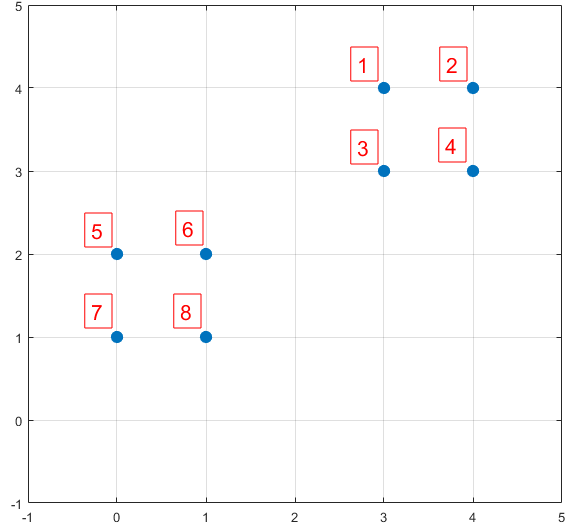图3. K-means++示例

假设经过图2的步骤一后6号点被选择为第一个初始聚类中心，那在进行步骤二时每个样本的D(x)和被选择为第二个聚类中心的概率如下表所示：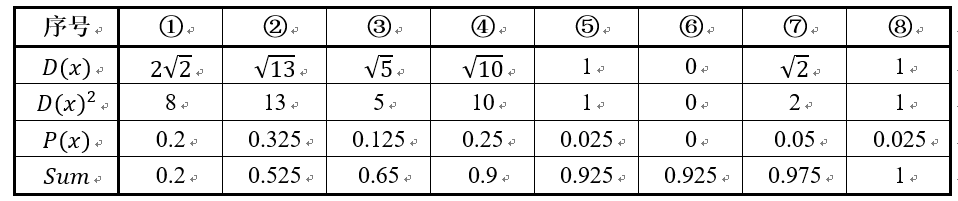其中的P(x)就是每个样本被选为下一个聚类中心的概率。最后一行的Sum是概率P(x)的累加和，用于轮盘法选择出第二个聚类中心。方法是随机产生出一个0~1之间的随机数，判断它属于哪个区间，那么该区间对应的序号就是被选择出来的第二个聚类中心了。例如1号点的区间为[0,0.2)，2号点的区间为[0.2, 0.525)。

从上表可以直观的看到第二个初始聚类中心是1号，2号，3号，4号中的一个的概率为0.9。而这4个点正好是离第一个初始聚类中心6号点较远的四个点。这也验证了K-means的改进思想：即离当前已有聚类中心较远的点有更大的概率被选为下一个聚类中心。

针对于python语言,已经有相关的官网有相关与K-means与K-means++相关算法的库，可以直接调用，但是当遇到多维特征向量的时候不容易直接调用，就比较难直接调用这些库实现，所以可以考虑对原理进行理解，自己编写代码尝试一下，因为K-menas也算是比较简单的算法啦，写起来也不是十分困难。

借鉴到的文章：

https://www.cnblogs.com/yixuan-xu/p/6272208.html

Kmeans加权聚类和多维聚类可视化 - 知乎

展开全文算法 聚类
• Kmeansplusplus 带有可选 k-means++ 初始化的 K-means 实现，基于 John Aleshunas 的 k-means 多属性实现。 需要 C++11； 没有其他依赖。 通过在源目录中运行make编译。用法运行k-means++ [control file name]控制...C++
• k means clustering matlab code
• k-means算法是一种动态聚类算法，基本原理如下：首先预先定义分类数k，并随机或按一定的原则选取k个样品作为初始聚类中心；然后按照就近的原则将其余的样品进行归类，得出一个初始的分类方案，并计算各类别的...
• k-means算法是无监督的聚类算法，实现起来较为简单，k-means++可以理解为k-means的增强版，在初始化中心点的方式上比k-means更友好。 k-means原理 k-means的实现步骤如下： 从样本中随机选取k个点作为聚类中心点 ...

## 前言

k-means算法是无监督的聚类算法，实现起来较为简单，k-means++可以理解为k-means的增强版，在初始化中心点的方式上比k-means更友好。

## k-means原理

k-means的实现步骤如下：

1. 从样本中随机选取k个点作为聚类中心点
2. 对于任意一个样本点，求其到k个聚类中心的距离，然后，将样本点归类到距离最小的聚类中心，直到归类完所有的样本点（聚成k类）
3. 对每个聚类求平均值，然后将k个均值分别作为各自聚类新的中心点
4. 重复2、3步，直到中心点位置不在变化或者中心点的位置变化小于阈值

优点：

• 原理简单，实现起来比较容易
• 收敛速度较快，聚类效果较优

缺点：

• 初始中心点的选取具有随机性，可能会选取到不好的初始值。

## k-means++原理

k-means++是k-means的增强版，它初始选取的聚类中心点尽可能的分散开来，这样可以有效减少迭代次数，加快运算速度，实现步骤如下：

1. 从样本中随机选取一个点作为聚类中心
2. 计算每一个样本点到已选择的聚类中心的距离，用D(X)表示：D(X)越大，其被选取下一个聚类中心的概率就越大
3. 利用轮盘法的方式选出下一个聚类中心(D(X)越大，被选取聚类中心的概率就越大)
4. 重复步骤2，直到选出k个聚类中心
5. 选出k个聚类中心后，使用标准的k-means算法聚类

这里不得不说明一点，有的文献中把与已选择的聚类中心最大距离的点选作下一个中心点，这个说法是不太准确的，准的说是与已选择的聚类中心最大距离的点被选作下一个中心点的概率最大，但不一定就是改点，因为总是取最大也不太好（遇到特殊数据，比如有一个点离某个聚类所有点都很远）。

一般初始化部分，始终要给些随机。因为数据是随机的。

尽管计算初始点时花费了额外的时间，但是在迭代过程中，k-mean 本身能快速收敛，因此算法实际上降低了计算时间。

现在重点是利用轮盘法的方式选出下一个聚类中心，我们以一个例子说明K-means++是如何选取初始聚类中心的。

假如数据集中有8个样本，分布分布以及对应序号如下图所示：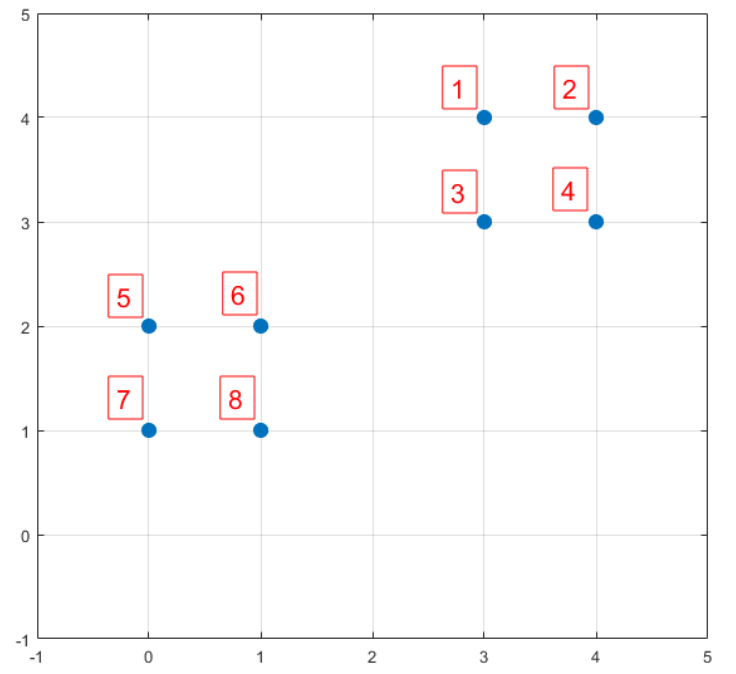我们先用 k-means++的步骤1选择6号点作为第一个聚类中心，然后进行第二步，计算每个样本点到已选择的聚类中心的距离D(X)，如下所示：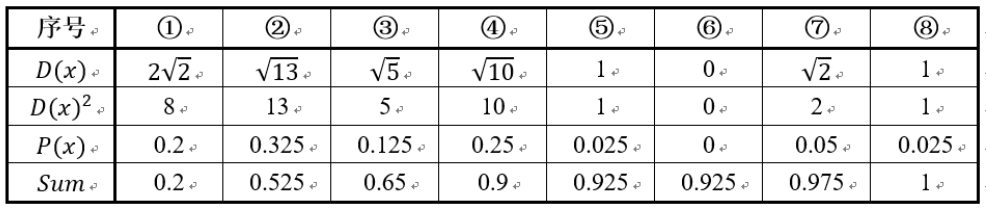• D(X)是每个样本点与所选取的聚类中心的距离(即第一个聚类中心)
• P(X)每个样本被选为下一个聚类中心的概率
• Sum是概率P(x)的累加和，用于轮盘法选择出第二个聚类中心。

然后执行 k-means++的第三步：利用轮盘法的方式选出下一个聚类中心，方法是随机产生出一个0~1之间的随机数，判断它属于哪个区间，那么该区间对应的序号就是被选择出来的第二个聚类中心了

在上图1号点区间为[0,0.2)，2号点的区间为[0.2, 0.525)，4号点的区间为[0.65,0.9)

从上表可以直观的看到，1号，2号，3号，4号总的概率之和为0.9，这4个点正好是离第一个初始聚类中心(即6号点)较远的四个点，因此选取的第二个聚类中心大概率会落在这4个点中的一个，其中2号点被选作为下一个聚类中心的概率最大。

## k-means及k-means++代码实现

这里选择的中心点是样本的特征(不是索引)，这样做是为了方便计算，选择的聚类点(中心点周围的点)是样本的索引。

k-means实现

# 定义欧式距离
import numpy as np
def get_distance(x1, x2):
return np.sqrt(np.sum(np.square(x1-x2)))

import random
# 定义中心初始化函数，中心点选择的是样本特征
def center_init(k, X):
n_samples, n_features = X.shape
centers = np.zeros((k, n_features))
selected_centers_index = []
for i in range(k):
# 每一次循环随机选择一个类别中心,判断不让centers重复
sel_index = random.choice(list(set(range(n_samples))-set(selected_centers_index)))
centers[i] = X[sel_index]
selected_centers_index.append(sel_index)
return centers

# 判断一个样本点离哪个中心点近， 返回的是该中心点的索引
## 比如有三个中心点，返回的是0，1，2
def closest_center(sample, centers):
closest_i = 0
closest_dist = float('inf')
for i, c in enumerate(centers):
# 根据欧式距离判断，选择最小距离的中心点所属类别
distance = get_distance(sample, c)
if distance < closest_dist:
closest_i = i
closest_dist = distance
return closest_i

# 定义构建聚类的过程
# 每一个聚类存的内容是样本的索引，即对样本索引进行聚类，方便操作
def create_clusters(centers, k, X):
clusters = [[] for _ in range(k)]
for sample_i, sample in enumerate(X):
# 将样本划分到最近的类别区域
center_i = closest_center(sample, centers)
# 存放样本的索引
clusters[center_i].append(sample_i)
return clusters

# 根据上一步聚类结果计算新的中心点
def calculate_new_centers(clusters, k, X):
n_samples, n_features = X.shape
centers = np.zeros((k, n_features))
# 以当前每个类样本的均值为新的中心点
for i, cluster in enumerate(clusters):  # cluster为分类后每一类的索引
new_center = np.mean(X[cluster], axis=0) # 按列求平均值
centers[i] = new_center
return centers

# 获取每个样本所属的聚类类别
def get_cluster_labels(clusters, X):
y_pred = np.zeros(np.shape(X))
for cluster_i, cluster in enumerate(clusters):
for sample_i in cluster:
y_pred[sample_i] = cluster_i
#print('把样本{}归到{}类'.format(sample_i,cluster_i))
return y_pred

# 根据上述各流程定义kmeans算法流程
def Mykmeans(X, k, max_iterations,init):
# 1.初始化中心点
if init == 'kmeans':
centers = center_init(k, X)
else: centers = get_kmeansplus_centers(k, X)
# 遍历迭代求解
for _ in range(max_iterations):
# 2.根据当前中心点进行聚类
clusters = create_clusters(centers, k, X)
# 保存当前中心点
pre_centers = centers
# 3.根据聚类结果计算新的中心点
new_centers = calculate_new_centers(clusters, k, X)
# 4.设定收敛条件为中心点是否发生变化
diff = new_centers - pre_centers
# 说明中心点没有变化，停止更新
if diff.sum() == 0:
break
# 返回最终的聚类标签
return get_cluster_labels(clusters, X)

# 测试执行
X = np.array([[0,2],[0,0],[1,0],[5,0],[5,2]])
# 设定聚类类别为2个，最大迭代次数为10次
labels = Mykmeans(X, k = 2, max_iterations = 10,init = 'kmeans')
# 打印每个样本所属的类别标签
print("最后分类结果",labels)
## 输出为  [1. 1. 1. 0. 0.]

# 使用sklearn验证
from sklearn.cluster import KMeans
X = np.array([[0,2],[0,0],[1,0],[5,0],[5,2]])
kmeans = KMeans(n_clusters=2,init = 'random').fit(X)
# 由于center的随机性，结果可能不一样
print(kmeans.labels_)


k-means++实现

## 得到kmean++中心点
def get_kmeansplus_centers(k, X):
n_samples, n_features = X.shape
init_one_center_i = np.random.choice(range(n_samples))
centers = []
centers.append(X[init_one_center_i])
dists = [ 0 for _ in range(n_samples)]

# 执行
for _ in range(k-1):
total = 0
for sample_i,sample in enumerate(X):
# 得到最短距离
closet_i = closest_center(sample,centers)
d = get_distance(X[closet_i],sample)
dists[sample_i] = d
total += d
total = total * np.random.random()

for sample_i,d in enumerate(dists): # 轮盘法选出下一个聚类中心
total -= d
if total > 0:
continue
# 选取新的中心点
centers.append(X[sample_i])
break
return centers

X = np.array([[0,2],[0,0],[1,0],[5,0],[5,2]])
# 设定聚类类别为2个，最大迭代次数为10次
labels = Mykmeans(X, k = 2, max_iterations = 10,init = 'kmeans++')
print("最后分类结果",labels)
## 输出为  [1. 1. 1. 0. 0.]

# 使用sklearn验证
X = np.array([[0,2],[0,0],[1,0],[5,0],[5,2]])
kmeans = KMeans(n_clusters=2,init='k-means++').fit(X)
print(kmeans.labels_)

展开全文kmeans算法
• K-means与K-means++区别 K-means算法步骤 1、随机初始化K个聚类中心 2、计算每个样本与k个聚类中心的相似度，将样本划分到与之最相似的类中 3、计算划分到每个类别中所有样本特征的均值，并将该均值作为每个类别...算法 机器学习
• matlab实现kmeans聚类算法，利用matlab自带工具箱的函数
• 针对传统分层路由算法存在的分簇不均匀、簇头选举不合理以及数据传输形式单一等问题，提出基于K-means++的无线传感网改进分簇算法LEACH-KPP。首先在成簇阶段采用K-means++算法实现均匀分簇，随后在簇头选举阶段使用...
• K-means，K-means++方法详解-机器学习分类问题常见算法算法 机器学习
• ## 详解k-means++

万次阅读 2020-12-20 18:34:16
K-means与K-means++：原始K-means算法最开始随机选取数据集中K个点作为聚类中心，而K-means++按照如下的思想选取K个聚类中心：假设已经选取了n个初始聚类中心(0<n<K)，则在选取第n+1个聚类中心时：距离当前n个...聚类 算法 机器学习
• 一、K-Means聚类 1.概念： k-means algorithm算法: K-均值（K-Means）属于聚类算法，之所以称为K-均值是因为它把n个样本根据它们的属性分为k个簇（k < n），且每个簇的中心采用簇中所含值的均值计算而成。...机器学习 数据挖掘 聚类算法 人工智能
• K-means及K-means++原理相对简单，直接引用了下文中的内容： https://www.cnblogs.com/yixuan-xu/p/6272208.html 本文重点是基于python实现了K-means和K-means++算法，并做了测试。 一、K-means 二、K-means++...机器学习 算法 python
• ## 聚类之K-Means++算法

千次阅读 2020-07-04 13:57:02
本篇博客主要分析了K-Means算法的不足之处，并由此介绍了K-Means++算法，用Python源码和sklearn机器学习库两种方式将算法具体实现。机器学习 python 算法 聚类
• 头文件： from sklearn.model_selection import train_test_split from sklearn.cluster import KMeans from sklearn.datasets import make_blobs from sklearn import metrics ...k_means = KMeans(init='k-msklearn python
• K-means ...为了解决初始点及孤立点问题，改进K-means++ 实际上仅优化了选取中心这一问题 ①选择一个样本作为第一个中心②计算其余点到此样本的距离，越大那么选择它作为下一个聚类中心的概算法 聚类
• 文章目录引言K-meansK-means++ 引言 最近在做YOLOv3的项目，想了想有哪些方面可以优化的，其中一个想法就是聚类算法了。 YOLOv3本身是用K-means聚类出锚框的，但K-means算法本身具有一定的局限性，聚类结果容易受...聚类算法 聚类
• 基于DTW的kmeans聚类算法，开发环境为matlab
• 一、k-means 1、简述 K-Means是一种无监督学习算法。算法的思想很简单，对于给定的样本集，按照样本之间的距离大小，将样本集划分为K个簇。让簇内的点尽量紧密的连在一起，而让簇间的距离尽量的大。 2、K-means算法...
• 为了解决因为初始化的问题带来K-Means算法的问题，改进的K-Means算法即K-Means++算法被提出。 K-Means++算法主要是为了能够在聚类中心的选择过程中选择较优的聚类中心。 K-Means++算法基本思路： ●在数据集中随机...python 算法
• 用于分类的K-means++聚类 用K-means++扩充实现k-means聚类算法。 K-means++ clustering for classification K-means clustering algorithm implementation with k-means++ augmentation.matlab...